# Draw a graph with two demand curves – one that is fairly elastic (labeled De) and...

Draw a graph with two demand curves – one that is fairly elastic (labeled De) and one that is fairly inelastic (labeled Di). Draw a supply curve and label it S. Suppose this market sees an increase in the price of this good due to the imposition of a tax. Draw the new supply curve and label it S2. Compare the impact in the market of the shift in supply between the elastic demand curve and the inelastic demand curve. Which demand curve would create more deadweight loss in the market when supply shifts? How can elasticity be used to explain the difference in the size of deadweight loss?

Deadweight loss refers to the inefficiency created by the market forces of demand and supply while creating the situation on non equilibrium. So here more of deadweight loss is created by the graph where demand was fairly elastic as here the supply has increased causing disequilibrium in the economy.
Size of the deadweight loss will be minimum in case of fairly inelastic demand because here there no change either in demand of in supply. On the other hand deadweight loss is more in case of fairly ealstic demand as here the supply has increased.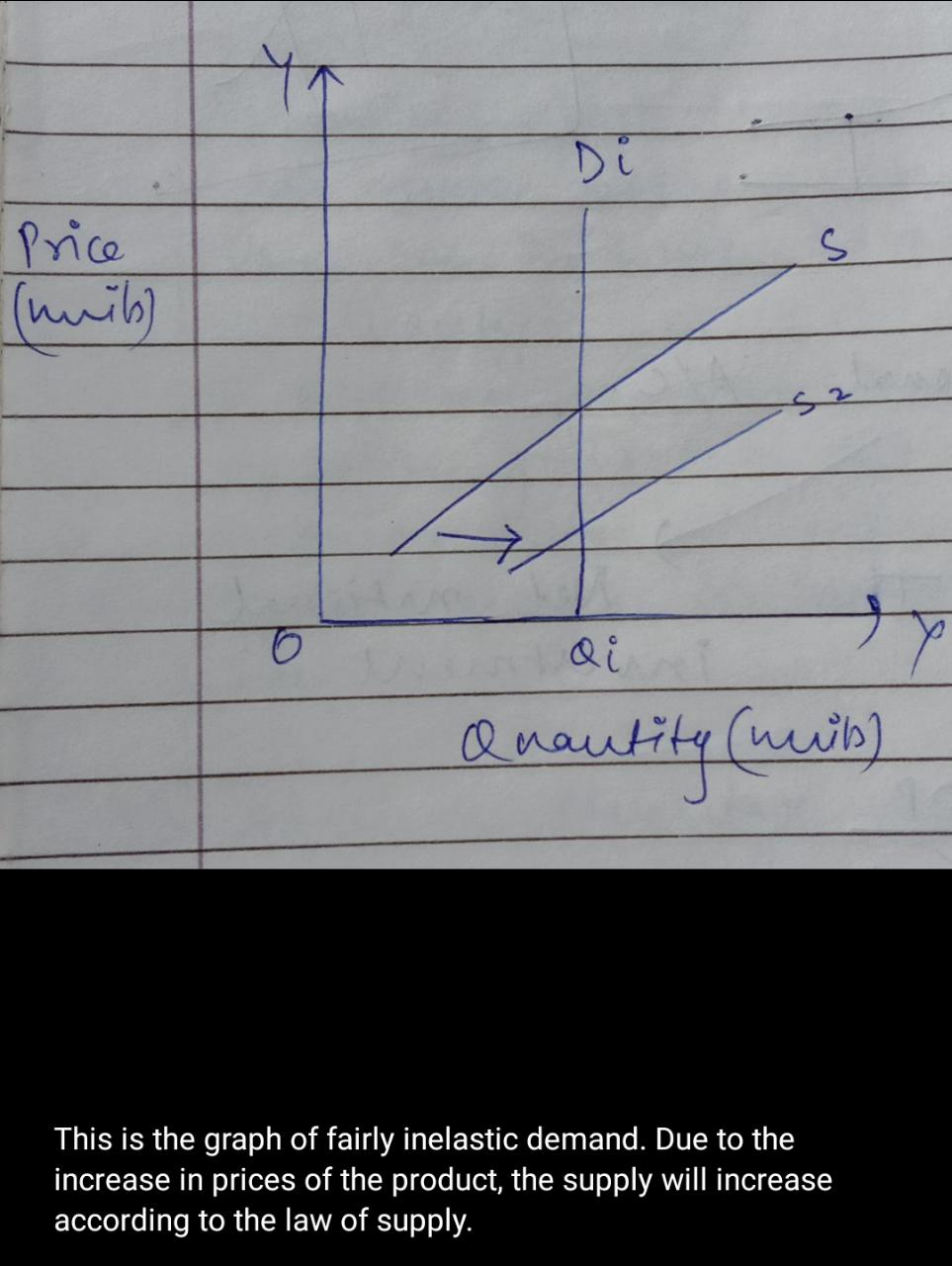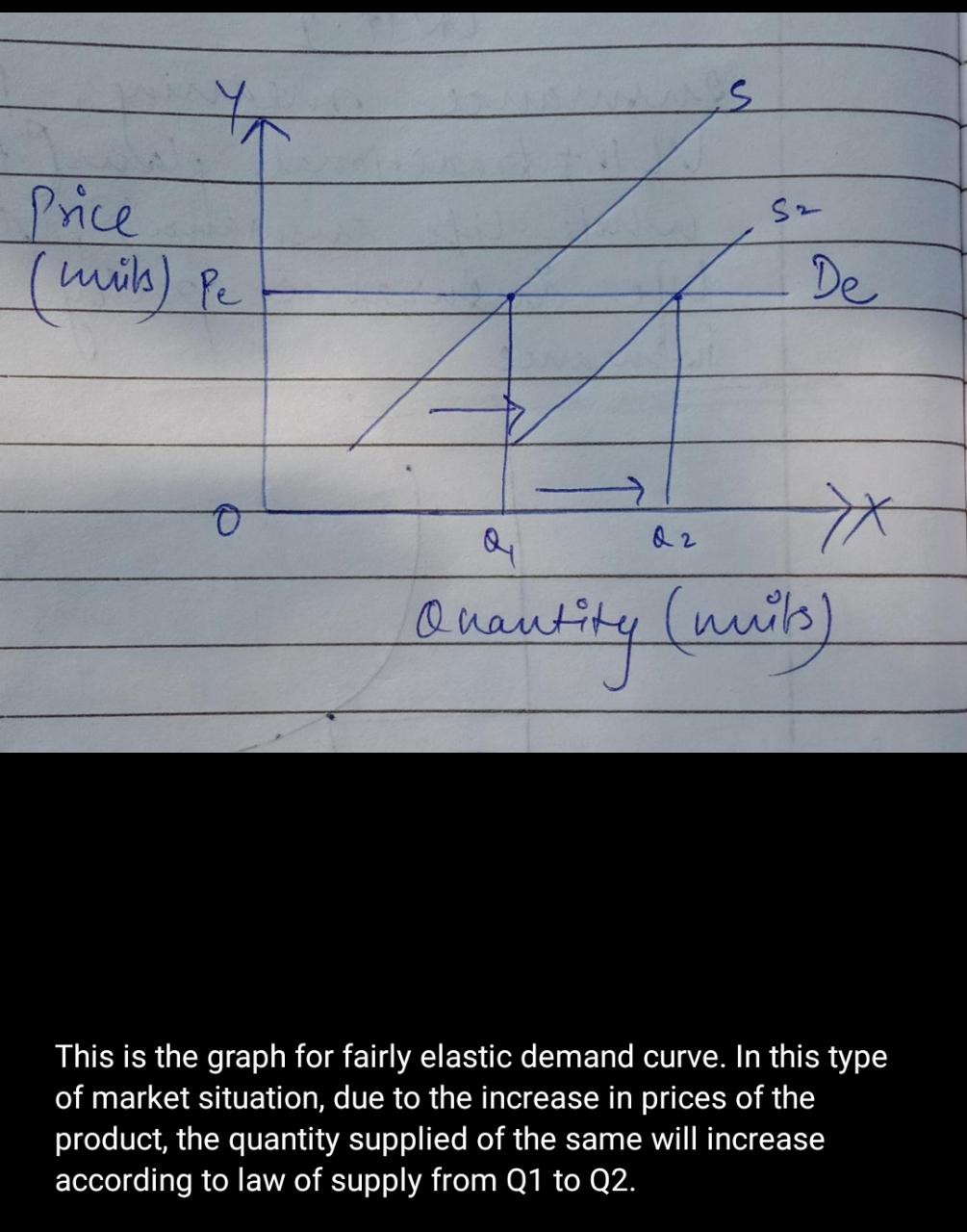##### Add Answer of: Draw a graph with two demand curves – one that is fairly elastic (labeled De) and...
Similar Homework Help Questions
• ### 5. If demand is elastic, will shifts in supply have a larger effect on equilibrium quantity...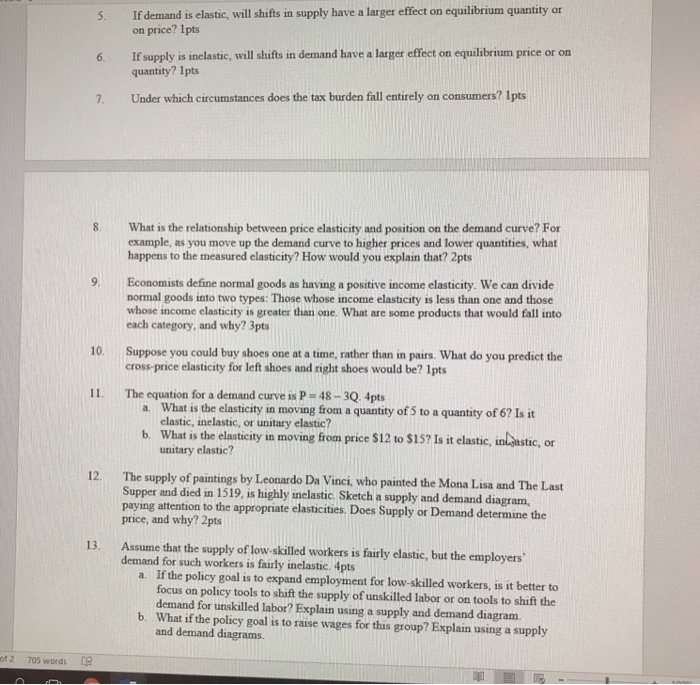5. If demand is elastic, will shifts in supply have a larger effect on equilibrium quantity or 6. If supply is inelastic, will shifts in demand have a larger effect on equilibrium price or on Under which circumstances does the tax burden fall entirely on consumers? İpts on price? Ipts quantity? 1pts 8. What is the relationship between price elasticity and position on the demand curve? For example, as you move up the demand curve to higher prices and lower...

• ### Please rank the follow curves as to how they would contribute to deadweight loss if a...

Please rank the follow curves as to how they would contribute to deadweight loss if a good that had this elasticity was taxed. Assume the supply curve has an elasticity equal to 1. Most deadweigh loss least deadweigh loss 1.a demand curve with price elastisity of demand equal to 100 2. a demand curve with price elastisity of demand equal to 0.4 3. a demand curve that is perfectly inelastic 4. a demand curve that is unit elastic

• ### Supply curves can have varying degrees of price elasticity. Show graphically a perfectly elastic, a perfectly...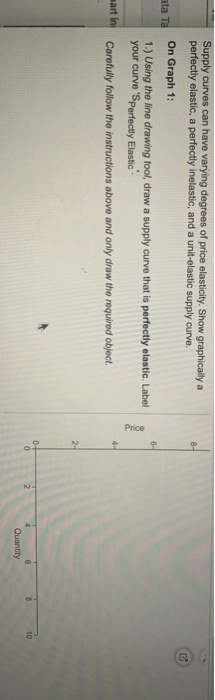Supply curves can have varying degrees of price elasticity. Show graphically a perfectly elastic, a perfectly inelastic, and a unit-elastic supply curve. ata Ta On Graph 1: 1.) Using the line drawing tool, draw a supply curve that is perfectly elastie. Label your curve 'Sperfectly Elastic Price martin Carefully follow the instructions above and only draw the required object Quantity

• ### Suppose these are the market demand and supply curves for hooded sweatshirts: Supply: P = 10...

Suppose these are the market demand and supply curves for hooded sweatshirts: Supply: P = 10 + 2QS Demand: P = 50−3QD (a) Sketch these two curves (that is, draw them, but don’t worry about numerical accuracy). Calculate equilibrium price and quantity. Calculate equilibrium price and quantity. (b) Show on your graph the areas of consumer and producer surplus. Calculate consumer and producer surplus at the equilibrium from part a. (c) Calculate the price elasticity of demand when price changes...

• ### #5 75. The graphs below show the market demand and supply curves for a good in...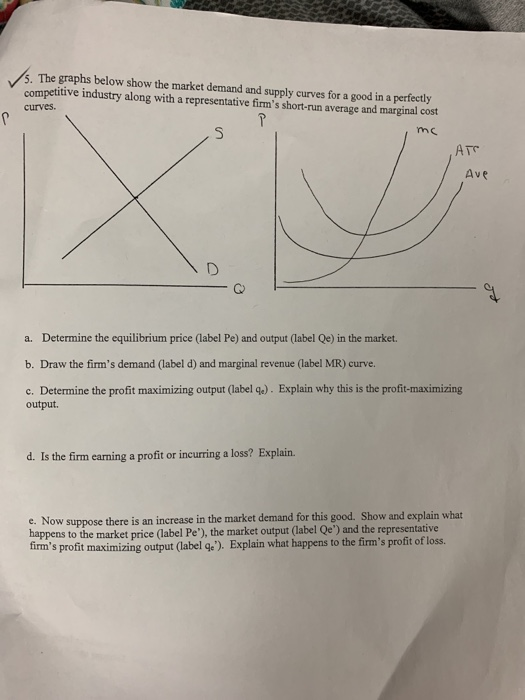#5 75. The graphs below show the market demand and supply curves for a good in a perfectly competitive industry along with a representative firm's short-run average and marginal cost curves. a. Determine the equilibrium price (label Pe) and output (label Qe) in the market. b. Draw the firm's demand (label d) and marginal revenue (label MR) curve. c. Determine the profit maximizing output (label 4). Explain why this is the profit-maximizing output d. Is the firm earning a profit...

• ### For these questions you are required to draw a properly labeled Supply/Demand graph with the initial...

For these questions you are required to draw a properly labeled Supply/Demand graph with the initial curves labeled S1 and D1, then show which curve shifts by drawing a second curve labeled S2 or D2. State whether the equilibrium price and quantity would increase, decrease, or stay the same. You may just draw the graphs by hand on a separate piece of paper and then take a picture of the page(s) and include them as attachments when you answer these...

• ### Q13 In a simple labor market model with perfect competition. 1. Draw a supply and demand,...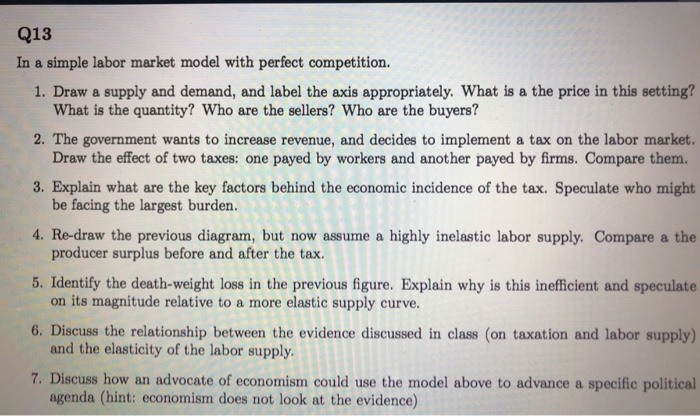Q13 In a simple labor market model with perfect competition. 1. Draw a supply and demand, and label the axis appropriately. What is a the price in this setting? What is the quantity? Who are the sellers? Who are the buyers? 2. The government wants to increase revenue, and decides to implement a tax on the labor market. Draw the effect of two taxes: one payed by workers and another payed by firms. Compare them. 3. Explain what are the...

• ### 9. On the graph below, using Supply and Demand, show what happens if a "large" country...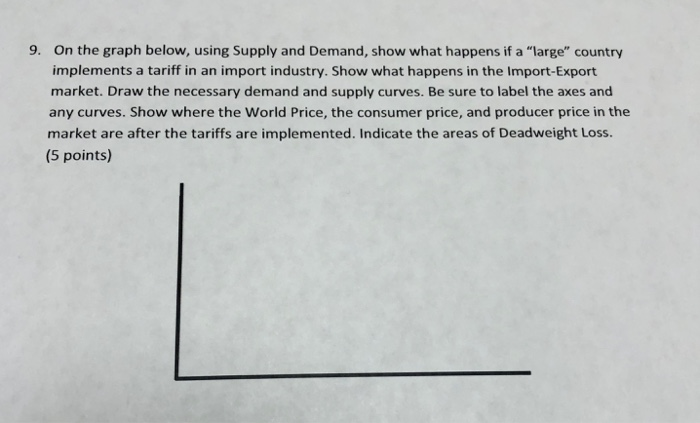9. On the graph below, using Supply and Demand, show what happens if a "large" country implements a tariff in an import industry. Show what happens in the Import-Export market. Draw the necessary demand and supply curves. Be sure to label the axes and any curves. Show where the World Price, the consumer price, and producer price in the market are after the tariffs are implemented. Indicate the areas of Deadweight Loss. (5 points)

• ### Understand the price elasticity of demand formula 2. Draw a perfectly elastic and perfectly inelastic demand...

Understand the price elasticity of demand formula 2. Draw a perfectly elastic and perfectly inelastic demand curve and label each 3. Be able to identify whether demand is elastic or inelastic given changes in quantity and price 4. Be able to calculate percentage change using the midpoint formula and be able to apply it to calculate the price elasticity of demand 5. Know the determinants of the price elasticity of demand and be able to identify how they change price...

• ### 8. On the graph below, using Supply and Demand, show what happens if a "large" country...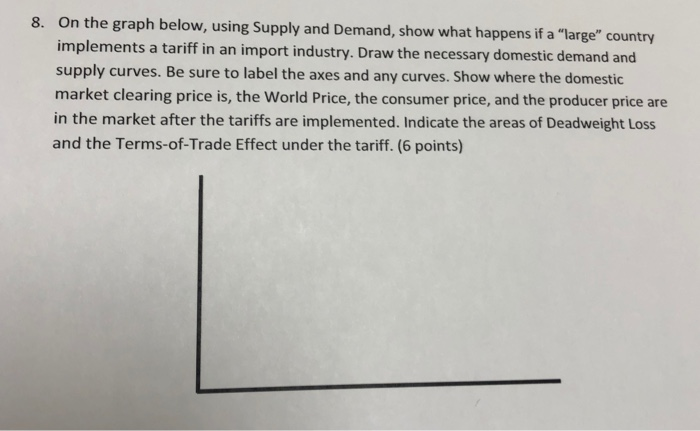8. On the graph below, using Supply and Demand, show what happens if a "large" country implements a tariff in an import industry. Draw the necessary domestic demand and supply curves. Be sure to label the axes and any curves. Show where the domestic market clearing price is, the World Price, the consumer price, and the producer price are in the market after the tariffs are implemented. Indicate the areas of Deadweight Loss and the Terms-of-Trade Effect under the tariff....

Need Online Homework Help?